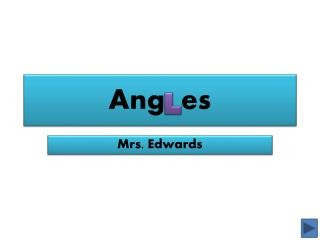DownloadDownload PresentationAng es

# Ang es

Download Presentation## Ang es

- - - - - - - - - - - - - - - - - - - - - - - - - - - E N D - - - - - - - - - - - - - - - - - - - - - - - - - - -
##### Presentation Transcript

1. Anges Mrs. Edwards

2. Contents What is an angle? Types of basic angles Where do we find angles? Student practice Core standards Citations *Click* on the angles to guide you through your learning

3. What is an angle? • An angle is when two straight half-lines (rays) meet together at an endpoint they share called a vertex. Vertex

4. Types of Basic Angles There are three basic angles we are going to learn. *Right Angles *Acute Angles *Obtuse Angles *Click on each angle to learn more

5. Right Angles A right angle comes together with one vertical line meeting one horizontal line at a 90 degree angle. It looks like this A square has 4 right angles!

6. Acute Angles Acute angles are like baby angles and are cute! They are always less than 90 degrees “A” for acute angle

7. Obtuse Angles Obtuse angles are wider than 90 degrees The “O” in obtuse reminds us it is Open wide

8. Where do we find angles? In our food On the In the Street Alphabet Everywhere!!! K

9. Can you find each kind of angle? Click to Reveal Angles

10. What angles can you find here? Click to Reveal Angles

11. Are there any angles here? Click to Reveal Angles

12. Can you find TWO of each angle? *Hint* Don’t forget to look in the letters Click to Reveal Angles

13. More angles practice!!! Here is a song about the three different angles we learned and how to remember them. For more angle fun visit: BBC Angles Activity Squirt Angles Game

14. Student Assignment Now that you have learned what the angles are and what they look like, your assignment is: Use a digital camera to make a Glogster poster about angles you found around your community. Be and let your personality show. Creative

15. Core Standards Math Standard 3- Students will describe and analyze attributes of two-dimensional shapes. Objective 1.d- Identify right angles in geometric figures, or in appropriate objects, and determine whether other angles are greater or less than a right angle. Intended Learning Outcomes: *Connect mathematical ideas within mathematics, to other disciplines, and to everyday experiences. *Represent mathematical ideas in a variety of ways

16. Citations Utah Lesson Plans. (2007, July 11). The angle tangle. Retrieved from http://www.uen.org/Lessonplan/ preview?LPid=18973